# Operational AmplifiersGet valuable resources straight to your inbox - sent out once per month

## What is an Operational Amplifier?

An operational amplifier (op amp) is an analog circuit block that takes a differential voltage input and produces a single-ended voltage output.

Op amps usually have three terminals: two high-impedance inputs and a low-impedance output port. The inverting input is denoted with a minus (-) sign, and the non-inverting input uses a positive (+) sign. Operational amplifiers work to amplify the voltage differential between the inputs, which is useful for a variety of analog functions including signal chain, power, and control applications.

## Operational Amplifier Clasifications

There are four ways to classify operational amplifiers:

• Voltage amplifiers take voltage in and produce a voltage at the output.
• Current amplifiers receive a current input and produce a current output.
• Transconductance amplifiers convert a voltage input to a current output.
• Transresistance amplifiers convert a current input and produces a voltage output.

Because most op amps are used for voltage amplification, this article will focus on voltage amplifiers.

## Operational Amplifiers: Key Characteristics and Parameters

There are many different important characteristics and parameters related to op amps (see Figure 1). These characteristics are described in greater detail below.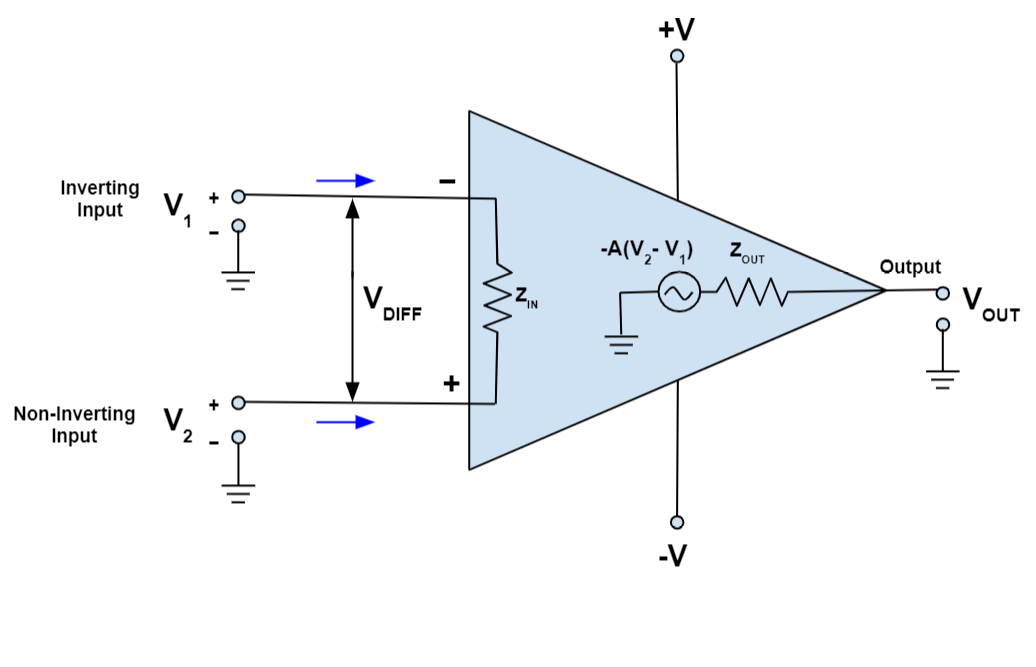Figure 1: Operational Amplifier Schematic

### Open-loop gain

Open-loop gain: The open-loop gain (“A” in Figure 1) of an operational amplifier is the measure of the gain achieved when there is no feedback implemented in the circuit. This means the feedback path, or loop, is open. An open-loop gain often must be exceedingly large (10,000+) to be useful in itself, except with voltage comparators.

Voltage comparators compare the input terminal voltages. Even with small voltage differentials, voltage comparators can drive the output to either the positive or negative rails. High open-loop gains are beneficial in closed-loop configurations, as they enable stable circuit behaviors across temperature, process, and signal variations.

### Input impedance

Another important characteristic of op amps is that they generally have high input impedance (“ZIN” in Figure 1). Input impedance is measured between the negative and positive input terminals, and its ideal value is infinity, which minimizes loading of the source. (In reality, there is a small current leakage.) Arranging the circuitry around an operational amplifier may significantly alter the effective input impedance for the source, so external components and feedback loops must be carefully configured. It is important to note that input impedance is not solely determined by the input DC resistance. Input capacitance can also influence circuit behavior, so that must be taken into consideration as well.

### Output impedance

An operational amplifier ideally has zero output impedance (“ZOUT” in Figure 1). However, the output impedance typically has a small value, which determines the amount of current it can drive, and how well it can operate as a voltage buffer.

### Frequency response and bandwidth (BW)

An ideal op amp would have an infinite bandwidth (BW), and would be able to maintain a high gain regardless of signal frequency. However, all operational amplifiers have a finite bandwidth, generally called the “-3dB point,” where the gain begins to roll as frequency increases. The gain of the amplifier then decreases at a rate of -20dB/decade while the frequency increases. Op amps with a higher BW have improved performance because they maintain higher gains at higher frequencies; however, this higher gain results in larger power consumption or increased cost.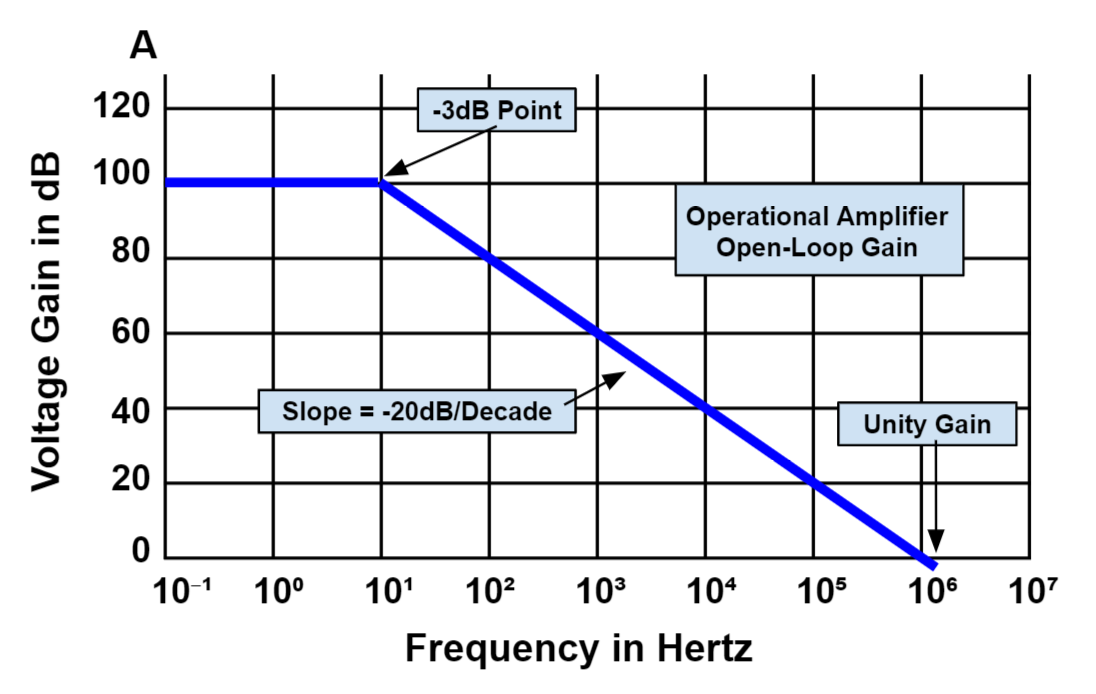Figure 2: Operational Amplifier Open-Loop Frequency Response Curve

### Gain bandwidth product (GBP)

As the name suggests, GBP is a product of the amplifier’s gain and bandwidth. GBP is a constant value across the curve, and can be calculated with Equation (1):

$$GBP = Gain x Bandwidth = A x BW$$

GBP is measured at the frequency point at which the operational amplifier’s gain reaches unity. This is useful because it allows the user to calculate the device’s open-loop gain at different frequencies. An operational amplifier’s GBP is generally a measure of its usefulness and performance, as op amps with a higher GBP can be used to achieve better performance at higher frequencies.

These are the major parameters to consider when selecting an operational amplifier in your design, but there are many other considerations that may influence your design, depending on the application and performance needs. Other common parameters include input offset voltage, noise, quiescent current, and supply voltages.

## Negative Feedback and Closed-Loop Gain

In an operational amplifier, negative feedback is implemented by feeding a portion of the output signal through an external feedback resistor and back to the inverting input (see Figure 3).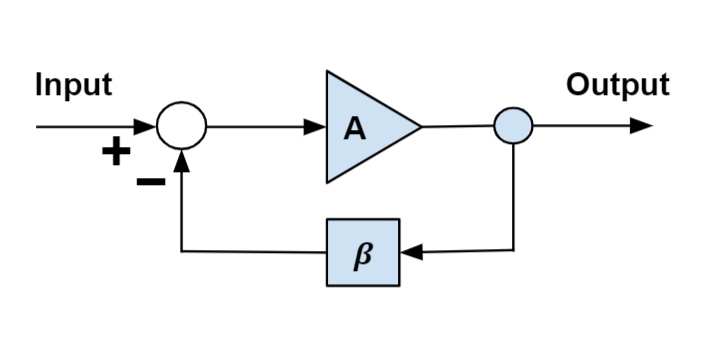Figure 3: Negative Feedback with Inverting Operational Amplifier

Negative feedback is used to stabilize the gain. By using a negative feedback, the closed-loop gain can be determined via external feedback components that can have higher accuracy compared to the operational amplifier’s internal components. This is because the internal op amp components may vary substantially due to process shifts, temperature changes, voltage changes, and other factors. The closed-loop gain can be calculated with Equation (2):

$$\frac {V_{OUT}}{V_{IN}} = \frac 1 f$$

## Operational Amplifiers: Advantages and Limitations

There are many advantages to using an operational amplifier. Operational amplifiers often come in the form of an IC, and are widely available, with countless selectable performance levels to meet every application’s needs. Op amps have a broad range of usages, and as such are a key building block in many analog applications — including filter designs, voltage buffers, comparator circuits, and many others. In addition, most companies provide simulation support, such as PSPICE models, for designers to validate their operational amplifier designs before building real designs.

The limitations to using operational amplifiers include the fact they are analog circuits, and require a designer that understands analog fundamentals such as loading, frequency response, and stability. It is not uncommon to design a seemingly simple op amp circuit, only to turn it on and find that it is oscillating. Due to some of the key parameters discussed earlier, the designer must understand how those parameters play into their design, which typically means the designer must have a moderate to high level of analog design experience.

## Operational Amplifier Configuration Topologies

There are several different op amp circuits, each differing in function. The most common topologies are described below.

### Voltage follower

The most basic operational amplifier circuit is a voltage follower (see Figure 4). This circuit does not generally require external components, and provides high input impedance and low output impedance, which makes it a useful buffer. Because the voltage input and output are equal, changes to the input produce equivalent changes to the output voltage.

$$V_{OUT} = V_{IN}$$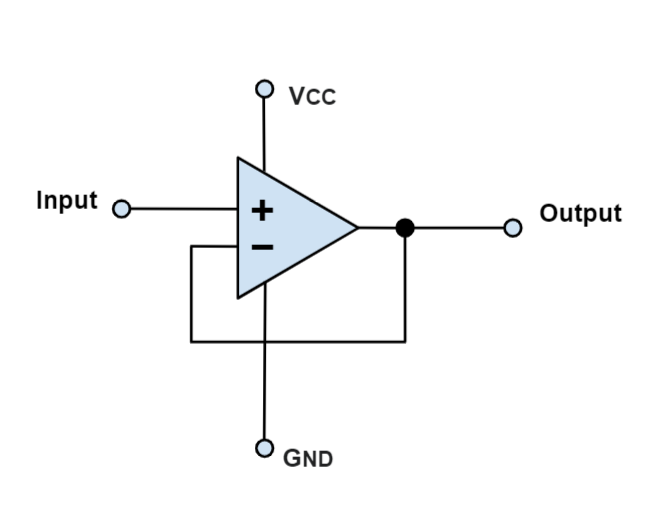Figure 4: Voltage Follower

The most common op amp used in electronic devices are voltage amplifiers, which increase the output voltage magnitude. Inverting and non-inverting configurations are the two most common amplifier configurations. Both of these topologies are closed-loop (meaning that there is feedback from the output back to the input terminals), and thus voltage gain is set by a ratio of the two resistors.

### Inverting operational amplifier

In inverting operational amplifiers, the op amp forces the negative terminal to equal the positive terminal, which is commonly ground. Therefore, the input current is determined by the VIN / R1 ratio (see Figure 5).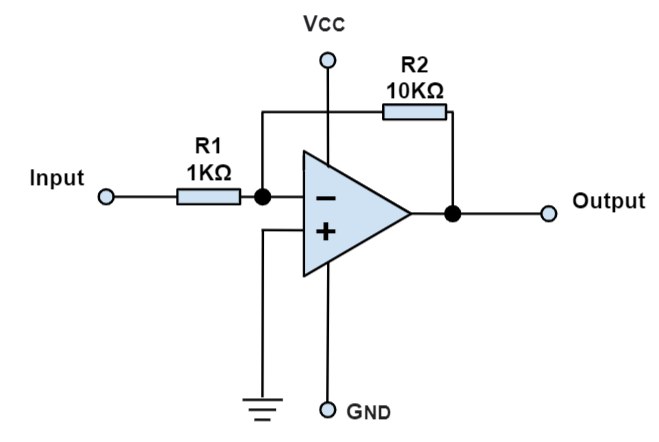Figure 5: Inverting Operational Amplifier

In this configuration, the same current flows through R2 to the output. Ideally, current does not flow into the operational amplifier’s negative terminal due to its high ZIN. The current flowing from the negative terminal through R2 creates an inverted voltage polarity with respect to VIN. This is why these op amps are labeled with an inverting configuration. Note that the op amp’s output can only swing between its positive and negative supplies, so creating a negative output voltage requires an op amp with a negative supply rail. VOUT can be calculated with Equation (3):

$$V_{OUT} = - \left({R_2} \over {R_1}\right) x V_{IN}$$

### Non-inverting operational amplifier

In a non-inverting amplifier circuit, the input signal from the source is connected to the non-inverting (+) terminal (see Figure 6).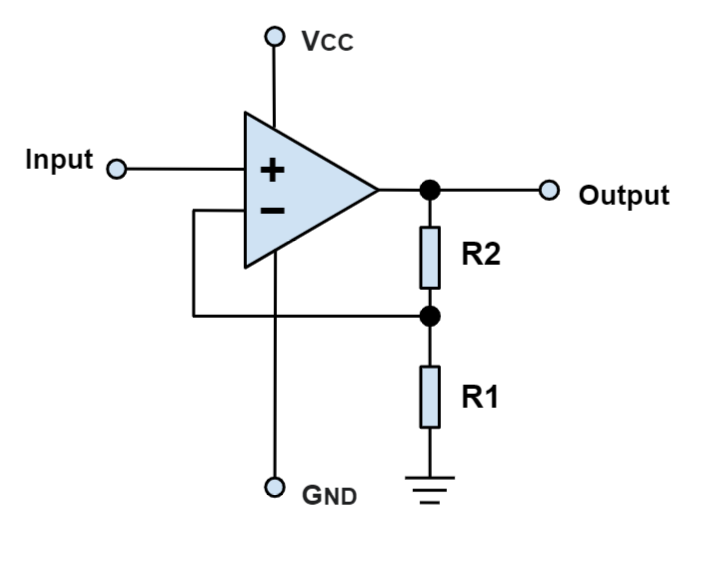Figure 6: Non-Inverting Operational Amplifier

The operational amplifier forces the inverting (-) terminal voltage to equal the input voltage, which creates a current flow through the feedback resistors. The output voltage is always in phase with the input voltage, which is why this topology is known as non-inverting. Note that with a non-inverting amplifier, the voltage gain is always greater than 1, which is not always the case with the inverting configurations. VOUT can be calculated with Equation (4):

$$V_{OUT} = (1 + \frac {R_2}{R_1}) \times V_{IN}$$

### Voltage comparator

An operational amplifier voltage comparator compares voltage inputs, and drives the output to the supply rail of whichever input is higher. This configuration is considered open-loop operation because there is no feedback. Voltage comparators have the benefit of operating much faster than the closed-loop topologies discussed above (see Figure 7).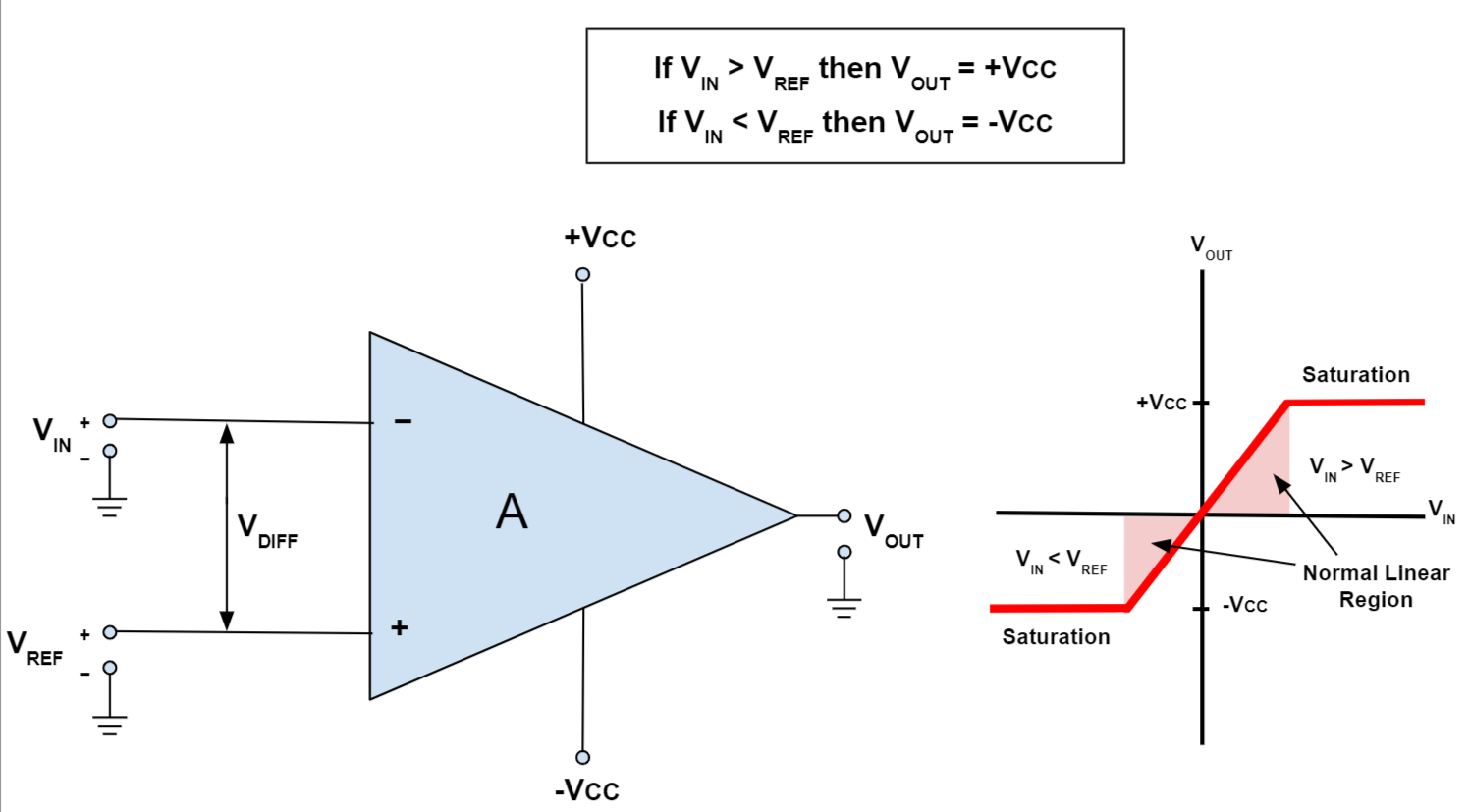Figure 7: Voltage Comparator

## How to Choose an Operational Amplifier for Your Application

The section below discusses certain considerations when selecting the proper operational amplifier for your application.

Firstly, choose an op amp that can support your expected operating voltage range. This information can be obtained by looking at the amplifier’s power supply voltages. The supply voltages will likely either be VDD (+) and ground (single supply), or the amplifier may be able to support both a positive and negative supply. A negative supply is useful if the output needs to support negative voltages.

Secondly, consider the amplifier’s GBP. If your application needs to support higher frequencies, or requires a higher performance and reduced distortion, consider op amps with higher GBPs.

One should also consider the power consumption, as certain applications may require low-power operation. The recommended power requirements can typically be found in the parts datasheet, and are usually listed as supply current and power consumption. Power consumption can also be estimated from the product of the supply current and supply voltage. Generally, op amps with lower supply currents have lower GBP, and correspond with lower circuit performance.

For applications that require higher accuracy, the designer should pay special attention to the amplifier’s input offset voltage, as this voltage leads to an offset in the amplifier's output voltage.

## Summary

Operational amplifiers are widely used in many analog and power applications. The benefits of using an op amp are that they are generally widely understood, well-documented and supported, and are fairly easy to use and implement. Op amps are useful for many applications, such as voltage buffers, creating analog filters, and threshold detectors. With a greater understanding of key parameters and common topologies related to operational amplifiers, you can begin implementing them in your circuits.

### _________________________

Did you find this interesting? Get valuable resources straight to your inbox - sent out once per month!

Get technical support